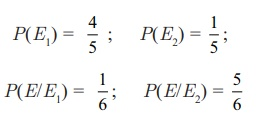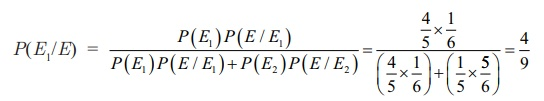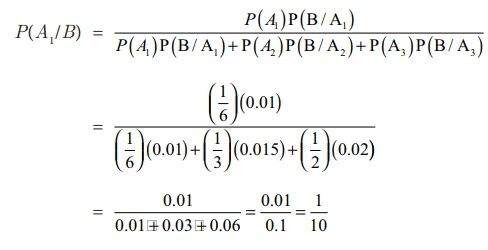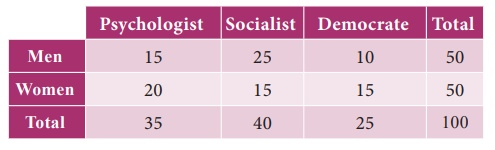Home | | Business Maths 11th std | Baye‚Äôs Theorem

# Baye‚Äôs Theorem

Solved Example Problems and Questions

Baye‚Äôs Theorem

If E1 , E2 , E3 ..., En are a set of n mutually exclusive and collectively exhaustive events with P(Ei) ‚âÝ 0 (i = 1,2,3‚Ä¶, n), then for any arbitrary event A which is associated with sample space S = Ui=1n   Ei such that P(A) > 0, we haveExample 8.31

Bag I contains 3 red and 4 blue balls while another Bag II contains 5 red and 6 blue balls. One ball is drawn at random from one of the bags and it is found to be red. Find the probability that it was drawn from second Bag.

Solution

Let E1 be the event of choosing the first bag, E2 the event of choosing the second bag and A be the events of drawing a red ball. Then P(E1) = P(E2) = ¬Ω

Also    P(A/E1) = P(drawing a red ball from Bag I) = 3/ 7

And    P(A/E2) = P(drawing a red ball from Bag II) = 5 /11

Now, the probability of drawing a ball from Bag II, being given that it is red, is P(E2/A).

By using Baye‚Äôs theorem, we haveExample 8.32

X speaks truth 4 out of 5 times. A die is thrown. He reports that there is a six. What is the chance that actually there was a six?

Solution:

Let us define the following events.

E1  : X speaks truth;

E2  : X tells a lie;

E : X reports a six;

From the data given in the problem, we haveThe required probability that actually there was six (by Bayes theorem) is P(E1) P(E/E1)P(E1) P(E/E1)+ P(E2) P(E/E2)

Example 8.33

A factory has 3 machines A1, A2, A3 producing 1000, 2000, 3000 screws per day respectively. A1 produces 1% defectives, A2 produces 1.5% and A3 produces 2% defectives. A screw is chosen at random at the end of a day and found defective. What is the probability that it comes from machines A1?

Solution:Let B be the event that the chosen screw is defective

‚à¥P(B/A1) = P(that defective screw from the machine A1) = 0.01

P(B/A2) = P(that defective screw from the machine A2) = 0.015 and

P(B/A3) = P(that defective screw from the machine A3) = 0.02

We have to find P(A1/B)

Hence by Baye‚Äôs theorem, we getExercise 8.2

1. A family has two children. What is the probability that both the children are girls given that at least one of them is a girl?

2. A die is thrown twice and the sum of the number appearing is observed to be 6. What is the conditional probability that the number 4 has appeared at least once?

3. An unbiased die is thrown twice. Let the event A be odd number on the first throw and B the event odd number on the second throw. Check whether A and B events are independent.

4. Probability of solving specific problem independently by A and B are ¬Ω and 1/3 respectively.  If both try to solve the problem independently, find the probability that the problem is

(i) solved

(ii) exactly one of them solves the problem

5. Suppose one person is selected at random from a group of 100 persons are given in the followingWhat is the probability that the man selected is a Psychologist?

6. Two urns contains the set of balls as given in the following tableOne ball is drawn from each urn and find the probability that

(i)  both balls are red

(ii)  both balls are of the same colour.

7. Bag I contains 3 Red and 4 Black balls while another Bag II contains 5 Red and 6 Black balls. One ball is drawn at random from one of the bags and it is found to be red. Find the probability that it was drawn from Bag I.

8. The first of three urns contains 7 White and 10 Black balls, the second contains 5 White and 12 Black balls and third contains 17 White balls and no Black ball. A person chooses an urn at random and draws a ball from it. And the ball is found to be White. Find the probabilities that the ball comes from

(i)  the first urn

(ii)  the second urn

(iii)  the third urn

9. Three boxes B1 , B2 , B3 contain lamp bulbs some of which are defective. The defective proportions in box B1, box B2 and box B3 are respectively 1/2 , 1/8 and 3/4 . A box is selected at random and a bulb drawn from it. If the selected bulb is found to be defective, what is the probability that box B1 was selected?

10. Three horses A, B, C are in race. A is twice as likely to win as B and B is twice as likely to win as C. What are their respective probabilities of winning?

11. A die is thrown. Find the probability of getting

(i) a prime number

(ii) a number greater than or equal to 3

12. Ten cards numbered 1 to 10 are placed in a box, mixed up thoroughly and then one card is drawn randomly. If it is known that the number on the drawn card is more than 4. What is the probability that it is an even number?

13. There are 1000 students in a school out of which 450 are girls. It is known that out of 450, 20% of the girls studying in class XI. A student is randomly selected from 1000 students. What is the probability that the selected student is from class XI given that the selected student is a girl?

14. From a pack of 52 cards, two cards are drawn at random. Find the probability that one is a king and the other is a queen.

15. A card is drawn from a pack of playing cards and then another card is drawn without the first being replaced. What is the probability of drawing

(i) two aces